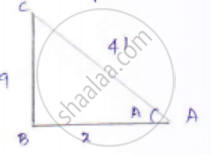Share

# If Sin a = 9/41 Compute Cos 𝐴 𝑎𝑛𝑑 Tan 𝐴 - Mathematics

Course

#### Question

If sin A = 9/41 compute cos 𝐴 𝑎𝑛𝑑 tan 𝐴

#### Solution

sin A = 9/41

sin A = "𝑜𝑝𝑝𝑜𝑠𝑖𝑡𝑒 𝑠𝑖𝑑𝑒"/"𝑎𝑑𝑗𝑎𝑐𝑒𝑛𝑡 𝑠𝑖𝑑𝑒" = 9/41

Consider the right-angled triangle ABC,Let x be the adjacent side

By applying Pythagorean

𝐴𝐶2 = 𝐴𝐵2 + 𝐵𝐶2

412 = 122 + 92

𝑥2 = 412 − 92

𝑥 = 40

cos A = "𝑎𝑑𝑗𝑎𝑐𝑒𝑛𝑡 𝑠𝑖𝑑𝑒"/"ℎ𝑦𝑝𝑜𝑡𝑒𝑛𝑢𝑠𝑒" = 40/41

tan A = "𝑜𝑝𝑝𝑜𝑠𝑖𝑡𝑒 𝑠𝑖𝑑𝑒"/"𝐻𝑦𝑝𝑜𝑡𝑒𝑛𝑢𝑠𝑒 𝑠𝑖𝑑𝑒" = 9/40

Is there an error in this question or solution?